## 视频背景分离

if args.algo == 'MOG2':
backSub = cv.createBackgroundSubtractorMOG2()
else:
backSub = cv.createBackgroundSubtractorKNN()


### github / cvzone

CVzone 是一个计算机视觉包，可以让我们轻松运行像人脸检测、手部跟踪、姿势估计等，以及图像处理和其他 AI 功能。它的核心是使用 OpenCV 和 MediaPipe 库。

• 60 FPS Face Detection 脸部检测
• Hand Tracking 手势跟踪
• Pose Estimation 人体姿态跟踪
• Face Mesh Detection 面部网格检测
• Stack Images 图片堆叠
• Corner Rectangle 选框显示
• FPS 帧率显示

## 二值化

### 二元阈值法

ret, thresh1 = cv2.threshold(img, 170, 255, cv2.THRESH_BINARY)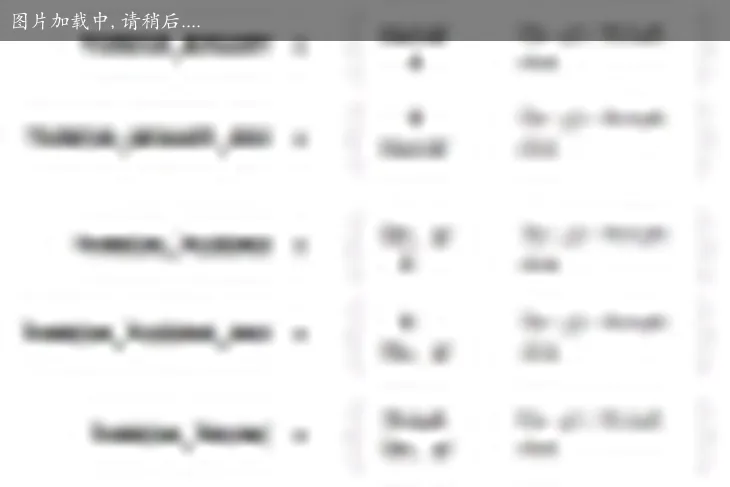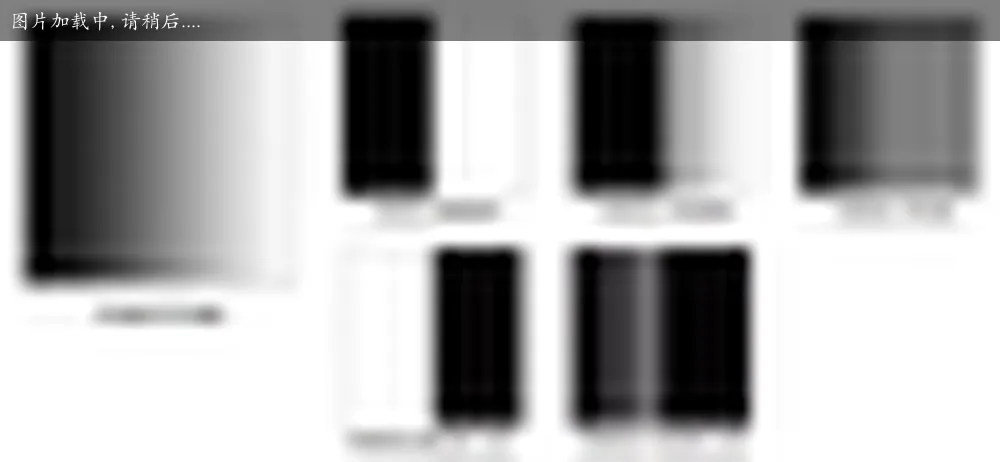### 自适应阈值

• 自适应阈值均值：阈值是平均值附近区域减去固定的 $C$。
• 自适应高斯阈值：阈值是邻域值减去常数 $C$ 的高斯加权总和。
th2 = cv2.adaptiveThreshold(img, 255, cv2.ADAPTIVE_THRESH_GAUSSIAN_C,
cv2.THRESH_BINARY, 11, 2)

/** @brief 自适应二值化
*@param _src      要二值化的灰度图
*@param _dst      二值化后的图
*@param maxValue  二值化后要设置的那个值
*@param type      二值化类型（CV_THRESH_BINARY 大于为最大值，CV_THRESH_BINARY_INV 小于为最大值）
*@param blockSize 块大小（奇数，大于 1）
*@param delta     差值（负值也可以）
*/
void cv::adaptiveThreshold(InputArray _src, OutputArray _dst, double maxValue,
int method, int type, int blockSize, double delta)
{
Mat src = _src.getMat();

// 原图必须是单通道无符号 8 位
CV_Assert(src.type() == CV_8UC1);

// 块大小必须大于 1，并且是奇数
CV_Assert(blockSize % 2 == 1 && blockSize > 1);
Size size = src.size();

// 构建与原图像相同的图像
_dst.create(size, src.type());
Mat dst = _dst.getMat();

if (maxValue < 0)
{
// 二值化后值小于 0，图像都为 0
dst = Scalar(0);
return;
}

// 用于比较的值
Mat mean;

if (src.data != dst.data)
mean = dst;

// 计算平均值作为比较值
boxFilter(src, mean, src.type(), Size(blockSize, blockSize),
Point(-1, -1), true, BORDER_REPLICATE);
// 计算高斯分布和作为比较值
GaussianBlur(src, mean, Size(blockSize, blockSize), 0, 0, BORDER_REPLICATE);
else

int i, j;

// 将 maxValue 夹到 [0,255] 的 uchar 范围区间，用作二值化后的值
uchar imaxval = saturate_cast<uchar>(maxValue);

// 根据二值化类型计算 delta 值
int idelta = type == THRESH_BINARY ? cvCeil(delta) : cvFloor(delta);

// 计算生成每个像素差对应的值表格，以后查表就可以。但像素差范围为什么是 768，我确实认为 512 已经够了
uchar tab;

if (type == CV_THRESH_BINARY)
for (i = 0; i < 768; i++)
// i = src[j] - mean[j] + 255
// i - 255 > -idelta ? imaxval : 0
// = src[j] - mean[j] + 255 -255 > -idelta ? imaxval : 0
// = src[j] > mean[j] - idelta ? imaxval : 0
tab[i] = (uchar)(i - 255 > -idelta ? imaxval : 0);
else if (type == CV_THRESH_BINARY_INV)
for (i = 0; i < 768; i++)
// i = src[j] - mean[j] + 255
// i - 255 <= -idelta ? imaxval : 0
// = src[j] - mean[j] + 255 - 255 <= -idelta ? imaxval : 0
// = src[j] <= mean[j] - idelta ? imaxval : 0
tab[i] = (uchar)(i - 255 <= -idelta ? imaxval : 0);
else

// 如果连续，加速运算
if (src.isContinuous() && mean.isContinuous() && dst.isContinuous())
{
size.width *= size.height;
size.height = 1;
}

// 逐像素计算 src[j] - mean[j] + 255，并查表得到结果
for (i = 0; i < size.height; i++)
{
const uchar* sdata = src.data + src.step*i;
const uchar* mdata = mean.data + mean.step*i;
uchar* ddata = dst.data + dst.step*i;

for (j = 0; j < size.width; j++)
// 将 [-255, 255] 映射到 [0, 510] 然后查表
ddata[j] = tab[sdata[j] - mdata[j] + 255];
}
}


### Otsu's Binrisation

ret3, th1 = cv2.threshold(img, 0, 255, cv2.THRESH_BINARY + cv2.THRESH_OTSU)


## 降噪

dst = cv2.fastNlMeansDenoisingColored(img, None, 10, 10, 7, 21)

• cv2.fastNlMeansDenoising() - 使用单个灰度图像
• cv2.fastNlMeansDenoisingColored() - 使用彩色图像。
• cv2.fastNlMeansDenoisingMulti() - 用于在短时间内捕获的图像序列（灰度图像）
• cv2.fastNlMeansDenoisingColoredMulti() - 与上面相同，但用于彩色图像。

## 背景颜色检测

### 算法二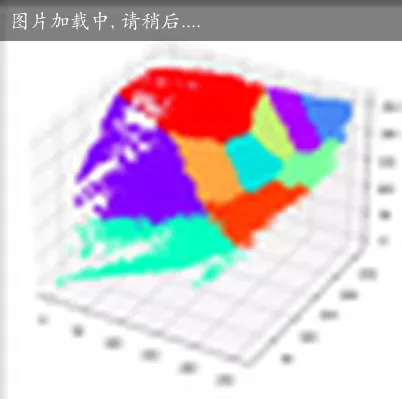code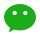### K-Means 算法描述

1. 适当选择 c 个类的初始中心；
2. 在第 k 次迭代中，对任意一个样本，求其到 c 各中心的距离，将该样本归到距离最短的那个中心所在的类；
3. 利用均值等方法更新该类的中心值；
4. 对于所有的 C 个聚类中心，如果利用（2）（3）的迭代法更新后，值保持不变，则迭代结束；否则继续迭代。

K-Means 算法试图找到使平凡误差准则函数最小的簇。 当潜在的簇形状是凸面的，簇与簇之间区别较明显，且簇大小相近时，其聚类结果较理想。

1. 聚类中心的个数 K 需要事先给定，但在实际中这个 K 值的选定是非常难以估计的，很多时候，事先并不知道给定的数据集应该分成多少个类别才最合适；
2. K-Means 需要人为地确定初始聚类中心，不同的初始聚类中心可能导致完全不同的聚类结果。（可以使用 K-Means++ 算法来解决）

## 使用深度学习生成模糊背景

code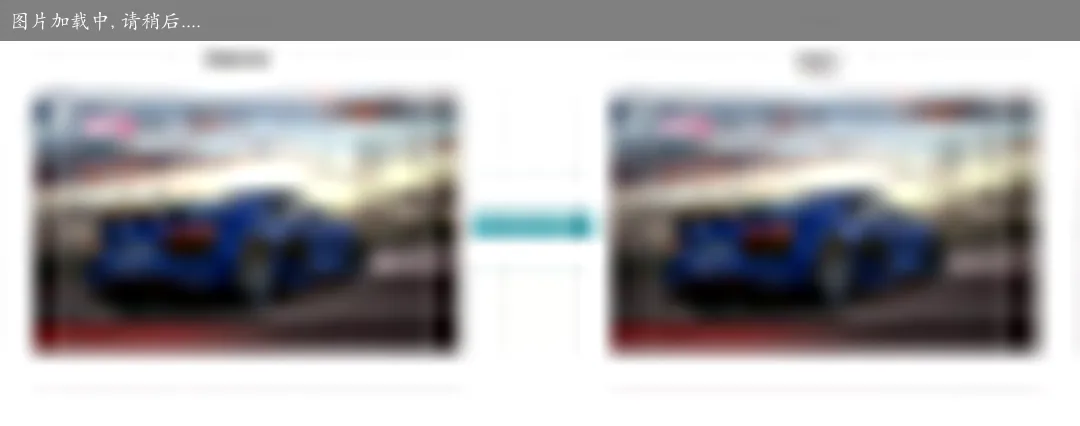If you have any questions or feedback, please reach out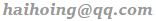.